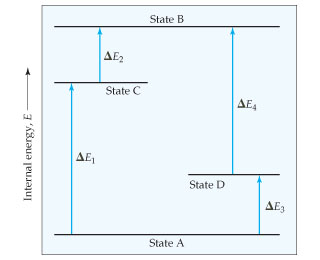# Problem: The diagram shows four states of a system, each with different internal energy, E. Suppose there is another state of the system, State E, and that its energy relative to State A is E = E1 + E4. Where would State E be on the diagram?

###### FREE Expert Solution

E = E1 + E → above state B###### Problem Details

The diagram shows four states of a system, each with different internal energy, E.Suppose there is another state of the system, State E, and that its energy relative to State A is E = E1 + E4. Where would State E be on the diagram?